## 7.20.2010

### SBG: Geometry Skills Draft

Last year I taught out of the McDougal Littell 2004 edition of Geometry Concepts and Skills. It was pretty watered down for a geometry course. This year I'm looking at the 2010 Glencoe Geometry book and it's a big jump. I will definitely be taking out a lot of these standards/sections.

Last year I did not teach similarity and one geometry class missed out on surface area and volume while the other missed out on circles and trig ratios. I don't want to talk about it. Anyway, I've never used this book before but so far I like the sequencing and for Illinois folk there are tons of PSAE problems, which is a plus.

I spent a few hours typing out these standards to build a concept list from and now my twitter friends are kind of talking me out of it. So, I may pull out my last year's Geo text and do those as well and combine. Or something.

Anyway, the list is huge and I made some notes and such along the way. So check them out and let me know what you're thinking.

All standards in red are ones I plan on chopping unless you say otherwise!

What should I eliminate, combine, rewrite, or rearrange?

I don't like how often it just says use, apply, recognize. Those words are somewhat vague. If I am a student how do I know that I used it right, applied the right thing, and recognized what I'm supposed to? It seems like they should be written differently in order to clearly assess.

Actually there are quite a few that I would really like to break down even more, but there's already 166 standards! That's ridiculous!

Geometry Glencoe Objectives

1 Identify and model points, lines, and planes
2 Identify intersecting lines and planes
3 Measure segments.
4 Calculate with measures
5 Determine precision of measurements
6 Determine accuracy of measurements
7 Find the distance between two points.
8 Find the midpoint of a segment.
9 Measure and classify angles.
10 Identify and use congruent angles and the bisector of an angle.
11 Identify and use special pairs of angles.
12 Identify perpendicular lines.
13 Identify and name polygons
14 Find perimeter, circumference, and area of two-dimensional figures.
15 Identify and name three-dimensional figures.
16 Find surface area and volume.
17 Make conjectures based on inductive reasoning.
18 Find counterexamples.
19 Determine truth values of negations, conjunctions, and disjunctions, and represent them using Venn diagrams.
20 Find counterexamples.
21 Analyze statements in if-then form.
22 Write the converse, inverse, and contrapositive of if-then statements.
23 Use the Law of Detachment
24 Use the Law of Syllogism.
25 Identify and use basic postulates about points, lines, and planes.
26 Write paragraph proofs.
27 Use algebra to write two-column proofs.
28 Use properties of equality to write geometric proofs.
29 Write proofs involving segment addition.
30 Write proofs involving segment congruence.
31 Write proofs involving supplementary and complementary angles.
32 Write proofs involving congruent and right angles.
33 Identify the relationships between two lines or two planes.
34 Name angle pairs formed by parallel lines and transversals.
35 Use theorems to determine the relationships between specific pairs of angles.
36 Use algebra to find angle measurements.
37 Find slopes of lines.
38 Use slope to identify parallel and perpendicular lines.
39 Write an equation of a line given information about the graph.
40 Solve problems by writing equations.
41 Recognize angle pairs that occur with parallel lines.
42 Prove that two lines are parallel using angle relationships.
43 Find the distance between a point and a line.
44 Find the distance between parallel lines.
45 Identify and classify triangles by angle measures.
46 Identify and classify triangles by side measures.
47 Apply the Triangle Angle-Sum Theorem.
48 Apply Exterior Angle Theorem.
49 Name and use corresponding parts of congruent polygons.
50 Prove triangles congruent using the definition of congruence.
51 Use the SSS Postulate to test for triangle congruence.
52 Use the SAS Postulate to test for triangle congruence.
53 Use the ASA Postulate to test for triangle congruence.
54 Use the AAS Postulate to test for triangle congruence.
55 Use properties of isosceles triangles.
56 Use properties of equilateral triangles.
57 Identify reflections, translations, and rotations.
58 Verify congruence after congruence transformation.
59 Position and label triangles for use in coordinate proofs. *Never done this before*
60 Write coordinate proofs. *Never done this before*
61 Identify and use perpendicular bisector in triangles.
62 Identify and use special bisectors in triangles.
63 Identify and use medians in triangles.
64 Identify and use altitudes in triangles.
65 Recognize and apply properties of inequalities to the measures of the angles of a triangle. *Not sure about this at all!*
66 Recognize and apply properties of inequalities to the relationships between the angles and sides of a triangle. *Not sure about this at all!*
67 Write indirect algebraic proofs. *NERVOUS!*
68 Write indirect geometric proofs. *NERVOUS!*
69 Use the Triangle Inequality Theorem to identify possible triangles.
70 Prove triangle relationships using the Triangle Inequality Theorem.
71 Apply the Hinge Theorem or its converse to make comparisons in two triangles. *NERVOUS!*
72 Prove triangle relationships using the Hinge Theorem or its converse. *NERVOUS!*
73 Find and use the sum of the measures of the interior angles of a polygon.
74 Find and use the sum of the measures of the exterior angles of a polygon.
75 Recognize and apply properties of the sides and angles of parallelograms.
76 Recognize and apply properties of the diagonals of parallelograms.
77 Recognize the conditions that ensure a quadrilateral is a parallelogram.
78 Prove that a set of points forms a parallelogram in the coordinate plane.
79 Recognize and apply properties of rectangles.
80 Determine whether parallelograms are rectangles.
81 Recognize and apply the properties of rhombi and squares.
82 Determine whether quadrilaterals are rectangles, rhombi, or squares.
83 Apply properties of trapezoids.
84 Apply properties of kites.
85 Write ratios.
86 Write and solve proportions.
87 Use proportions to identify similar polygons.
88 Solve problems using the properties of similar polygons.
89 Identify similar triangles using the AA Similarity Postulate and the SSS and SAS Similarity Theorem.
90 Use similar triangles to solve problems.
91 Use proportional parts within triangles.
92 Use proportional parts with parallel lines.
93 Recognize and use proportional relationships of corresponding angle bisectors, altitudes, and medians of similar triangles.
94 Use the Triangle Bisector Theorem. *Never done this before*
95 Identify similarity transformations. *Never done this before*
96 Verify similarity after a similarity transformation. *Never done this before*
97 Interpret scale models. *Never done this before*
98 Use scale factor to solve problems. *Never done this before*
99 Find the geometric mean between two numbers.
100 Solve problems involving relationships between parts of a right triangle and the altitude to its hypotenuse.
101 Use the Pythagorean Theorem.
102 Use the Converse of the Pythagorean Theorem.
103 Use the properties of 45-45-90 triangles.
104 Use the properties of 30-60-90 triangles.
105 Find trigonometric ratios using right triangles.
106 Use trigonometric ratios to find angle measures in right triangles.
107 Solve problems involving angles of elevation and depression.
108 Use angles of elevation and depression to find the distance between two objects.
109 Use the Law of Sines to solve triangles.
110 Use the Law of Cosines to solve triangles.
113 Draw reflections.
114 Draw reflections in the coordinate plane.
115 Draw translations.
116 Draw translations in the coordinate plane.
117 Draw rotations.
118 Draw rotations in the coordinate plane.
119 Draw glide reflections and other compositions of isometries in the coordinate plane.
120 Draw compositions of reflections in parallel and intersecting lines.
121 Identify line and rotational symmetries in two-dimensional figures.
122 Identify line and rotational symmetries in three-dimensional figures.
123 Draw dilations.
124 Draw dilations in the coordinate plane.
125 Identify and use parts of circles.
126 Solve problems involving the circumference of a circle.
127 Identify central angles, major arcs, minor arcs, and semicircles, and find their measures.
128 Find arc lengths .
129 Recognize and use relationships between arcs and chords.
130 Recognize and use relationships between arcs, chords, and diameters.
131 Find measures of inscribed angles.
132 Find measures of angles of inscribed polygons.
133 Use properties of tangents.
134 Solve problems involving circumscribed polygons.
135 Find measures of angle formed by lines intersecting on or inside a circle.
136 Find measures of angles formed by lines intersecting outside the circle.
137 Find measures of segments that intersect in the interior of a circle.
138 Find measures of segments that intersect in the exterior of a circle.
139 Write the equation of a circle.
140 Graph a circle on a coordinate plane.
141 Find perimeters and areas of parallelograms.
142 Find perimeters and areas of triangles.
143 Find areas of trapezoids.
144 Find areas of rhombi and kites.
145 Find areas of circles.
146 Find areas of sectors of circles.
147 Find areas of regular polygons.
148 Find areas of composite figures.
149 Find areas of similar figures by using scale factors.
150 Find scale factors or missing measures given the areas of similar figures.
151 Draw isometric views of three-dimensional figures. *This made me cry in college (the unhappy kind, not the Jesse Johnson kind)*
152 Investigate cross sections of three-dimensional figures.
153 Find lateral areas and surface areas of prisms.
154 Find lateral areas and surface areas of cylinders.
155 Find lateral areas and surface areas of pyramids.
156 Find lateral areas and surface areas of cones.
157 Find volumes of prisms.
158 Find volumes of cylinders.
159 Find volumes of pyramids.
160 Find volumes of cones.
161 Find surface areas of spheres.
162 Find volumes of spheres.
163 Describe sets of points on a sphere.
164 Compare and contrast Euclidean and spherical geometries.
165 Identify congruent or similar solids.
166 Use properties of similar solids.

There is one more chapter on probability and measurement but I really doubt I will even make it through these previous 12 chapters!

1.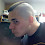Elissa: I think you might need to look for the forest from the trees here. How many standards would you be able to handle looking at, if you were the student? There's got to be a way to consolidate these into the bigger ideas that students need to learn. What is it about finding the areas of different figures that piques the same part of the brain? That's the standard.

2.I agree with Shawn that you need to cluster ideas, but I see nothing wrong with assessing 166 things. At one question each that is one question a day.

In fact, why not go for exactly as many assessments as days of instruction? The exit ticket each day could be one or two questions.

Having lots of little things to assess doesn't eliminate the need for clustering things into 10-15 units though.

3.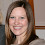That is a long list of things to assess. It appears to me that some of the ones you listed are things that students already have exposure to (find perimeters and area of parallelograms). Would it make more sense to scaffold the skills. What you really want students to do is find areas of composite figures. If a student can do that great. If not, you have the scaffold things you need to asses to bring them up to that point. Identify the prereq skills they need to successful. It just seems that you are trying to teach multiple years of material in one course. Another example I notice is Draw rotations. Your learning target is for students to be able to do it in the coordinate plane. Do you need to have it listed both ways?

4.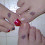I agree that most of your red statements can be eliminated. However, you need to check with other teachers in your state because some may be needed in your state. For example, #151 is required in my state and the Hinge Theorem is pretty common sense to most kids, although I'm not sure how much you need to do with it. Pretty much the 12 chapters you listed are the required topics for my state, but I do agree that some of these can be combined like #113/114 and what is the difference between #129 and 130?

When we created our list, we also went through every section of the book and looked at its objectives, but we also looked at the HW problems for that section and asked if there was a way we could clarify what the objective was or even if it was an objective that was vitally important or not so much. For example, what does #12 mean? How would you assess that? Is it a definition only? I think if you look at the problems in each section and match it up to the objective, you might get a clearer answer as to what in the world does this mean? Another example is #21 - what does analyze if-then form mean? Does it mean to identify the hypothesis and conclusion of an if-then statement? If so clarify that...

Also, depending on your state standards, you may find some that can be deleted and some that need to be added. In our state, #19 could be deleted, but I would need to add in "writing a biconditional statment".

Good luck!

5.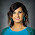Shawn,
I could cluster them but what's the point? I want them to be broken down clearly so that the student and I can immediately identify what they understand and what they don't. I could cluster and say "Write proofs" but how is that helpful?

gasstation,
We probably don't even have 166 instructional days!

6.Sarah,
I can definitely combine the 'in the coordinate planes' standards. As far as the area of composite figures, I see your point but I'm less sure of doing that. But I think you are right about some of the skills being prerequisites to others. Need to think about that.

MizT,
I don't know who to check with! That's why I'm asking you guys. Standards are very vague and it's not in the ACT Skills which is what we're assessed on so I'm at a loss. Thanks for pointing out looking at homework and such though, I hadn't thought about that.

7.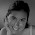I see several places where you could combine some standards. For example, why not combine 51-54? I mean, wouldn't an assessment of triangle congruence postulates involve correctly identifying which postulate is applicable in a given situation?

Also 139 & 140. In order to graph a circle (I assume from the equation) you would need to be comfortable with the equation. I see these as just inverse processes. Ability or inability with one implies ability or inability with the other.

Kind of the same idea with 149 & 150, 95 & 96.

That's what I've got for now.

8.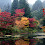I love geometry. I’ve never hidden that nerdy fact. However, I finally learned something about it this year – it’s really NOT all important. So here’s what I did for myself, and as scary as it was, it was freeing. I know not everyone can do this (but we’re lucky – the state doesn’t give us a list of “must covers” for each course).
There’s a LOT in geometry. So, I asked myself “what is enduring from geometry?” Meaning, what will the kids need in the next course, two courses from now, ACT, college, life, job, etc. from geometry. Oddly, the answers to each one were pretty similar. And I came up with my enduring list:
- Basic knowledge of points, lines, planes, segments, angles, shapes
- Basic knowledge of properties of triangles & quadrilaterals
- Parallel lines
- Area, Surface Area & volume
- Right triangle trig

Of course, there are a multitude of different skills & content pieces you can list under each topic, but the key is to limit yourself and aim for depth, not breadth. Here’s what I mean: should the kids know the triangle inequality theorem? Yup. They’ll need it again. It’s basic, and if you ask some adults, some might even remember it. Will they ever use Hinge theorem again? Hell no.

So here’s what I’m trying to say. From what I’ve read from you on twitter & your blog, your kids are coming in pretty low. They didn’t master the Algebra standards they needed to and now throwing 166 new standards at them will probably not yield a different result. So, maybe pick the most important ones and weave algebra everywhere. Example: you want them to remember the term bisector and what it means in context. Instead of this problem

http://img.skitch.com/20100723-xsnuq1eaifm9x6axngi5r4naag.jpg

Maybe try this one.

http://img.skitch.com/20100723-rc4qtr12nj7b7acj8gw57atern.jpg

Of even better, this (this could take your kids a while. And that’s ok! Makes ‘em think ☺ )

http://img.skitch.com/20100723-prh3xj5wt1act4u9damcfp44ik.jpg

A couple more suggestions (these are just IMHO)
- stay away from formal proof if your kids really are low. Instead, ask them the more powerful and thought-provoking questions – why does that work? Justify. Explain. Reason. Make a conjecture/conclusion, etc.
- stay away from having them memorize minor details (ie. Hinge theorem, circumcenters, incenters, etc, LOS, LOC, vectors, the million properties of circles, etc)
- I think the other commenters were trying to say that a list like this looks intimidating to students. In the beginning of the yr., you want to be upfront & honest with them. Giving them a list of learning targets is great, but seeing 166 of them will make them shut down before they’ve even had a chance to try. So maybe condense in a couple of units with a couple of broad LT to present to ss and then you can assess on whatever you want.
- Deeper, more “thinking” and discussion problems will make for a much more pleasant learning environment. Let kids get caught up in the depth and connections of problems rather than in trying to figure out which memorized theorem/property applies.

Just my 2 cents (although the length of this comment suggests 4 cents :-/ )

9.iTeach, I also prefer depth to breadth. I was with you until you tossed out vectors. The concept of vectors is more valuable than a lot of stuff you kept. I'd say that vectors are more valuable than right-angle trig or properties of quadrilaterals.

I recently was reading on Pat's Blog suggesting vectors as the basis for a lot of high-school math:
http://pballew.blogspot.com/2010/06/more-on-vectors-in-hs-curriculum.html

10.gasstationwithoutpumps, in the particular book she's working with, vectors are at the end of a Right-Triangle trig unit. It's an intense little section with a TON of information on vectors that seems to be very oddly placed. It's applying rt. triangle trig to vectors.
I both agree & disagree with you. I looked at Pat's blog - he said " think the integration of a few “15 minute vector asides” at a number of places each year". He doesn't suggest making vectors an entire big unit or learning target. I taught transformations using vectors to my honors classes last year & it made sense in our context. This list is for a prep-level course - I don't think adding vector learning targets is essential. Weaving basics here and there, yes.
We also have to remember something else - essentials for kids will be unfortunately be different from class to class, state to state, situation to situation and even level to level. It's the reality of an educational system with very different needs and demands from class to class. Depending on what set of students we have in front of us, we will each have our own opinions. Maybe common core is trying to unite us all... but that's a WHOLE other discussion! :)